# 1 Purpose

There are numerous programs out there for performing acoustic analysis, including several open-source options and R packages. For in-depth analysis of individual mammalian sounds it’s hard to beat PRAAT (batch processing is possible, but a bit tricky, because PRAAT uses its own, rather unusual scripting language). For bird sounds, a sophisticated tool is Sound Analysis Pro. In R, the most general-purpose acoustic toolkit is the seewave package. Soundgen builds upon the functionality of seewave, adding high-level functions for sound synthesis (see the vignette on sound synthesis), manipulation, and analysis.

Reasons to use `soundgen` for acoustic analysis might be:

1. User-friendly approach: a single call to the `analyzeFolder` function will give you a dataframe containing dozens of commonly used acoustic descriptors for each file in an entire folder. So if you’d rather get started with model-building without delving too deeply into acoustics, you are one line of code away from your dataset.
2. Flexible pitch tracking: soundgen uses several popular methods of pitch detection in parallel, followed by their integration and postprocessing. While the abundance of control parameters may initially seem daunting, for those who do wish to delve deeply this makes soundgen’s pitch tracker very versatile and offers a lot of power for high-precision analysis.
3. An interactive app for manual correction of pitch contours - `pitch_app()`.
4. Audio segmentation with in-built optimization: the tools for syllable segmentation and detection of energy bursts are fast and simple (based on smoothed intensity contours) but quite flexible. Control parameters can also be optimized automatically as long as you have a manually segmented training sample.
5. Additional specialized tools for acoustic analysis such as modulation spectra and self-similarity matrices.

Many of the large variety of existing tools for acoustic analysis were designed with a particular type of sound in mind, usually human speech or bird songs. Soundgen’s pitch tracker was written to analyze human non-linguistic vocalizations like screams and laughs. These sounds are much harsher and noisier than ordinary speech and stand much closer to the vocalizations of other mammals than to human speech. In addition, the original corpus (Anikin & Persson, 2017) was collected from online videos, so that both sampling rate and microphone settings varied tremendously. From the very beginning, the focus has thus been on developing a pitch tracker and a segmenting tool that would be robust to noise and recording conditions. This makes soundgen highly suitable for performing acoustic analysis of animal vocalizations. You can of course apply soundgen to speech, but note that it was not optimized for speech, unlike specialized phonetic software like Praat.

To summarize, you might want to look at soundgen’s tools for acoustic analysis if you are extracting a large number of acoustic predictors from a large number of audio files, for example:

• describing the vocal repertoire of a species (clustering),
• using machine learning for acoustic classification,
• comparing different classes of sounds in terms of specific acoustic predictors.

The most relevant functions are:

• `analyze`, `analyzeFolder`: extracts a number of acoustic predictors such as pitch, harmonics-to-noise ratio, mean frequency, peak frequency, formants, etc. The output can be a summary per file, with each variable presented as mean / median / SD, or you can obtain detailed statistics per STFT frame.
• `pitch_app`: opens a shiny app in a web browser for manually correcting and exporting the pitch contours extracted by `analyze`.
• `segment`, `segmentFolder`: finds syllables and bursts of energy using the amplitude envelope
• `optimizePars`: optimizes control parameters of `segment` or `analyze` aiming to reproduce manual segmentation of a training sample
• `ssm`: produces a self-similarity matrix and calculates novelty as an alternative method of audio segmentation
• `getLoudness`, `getLoudnessFolder`: estimates the subjective loudness per STFT frame, in sone
• `modulationSpectrum`, `modulationSpectrumFolder`: calculates a joint temporal-spectral modulation spectrum and a measure of acoustic roughness

TIP Soundgen’s functions for acoustic analysis are not meant to be exhaustive. MFCC extraction is readily available in R (e.g., with `tuneR::melfcc`), so there was no need to duplicate it in soundgen. Linear predictive coding (LPC) is also implemented in R (see `phonTools::lpc` and `phonTools::findformants`). As a convenience, `soundgen::analyze` shows the output of `phonTools::findformants`, but for serious formant analysis you might want to use an interactive program like PRAAT and check everything manually. A good approach may be to start with `soundgen::analyze` to get a table of many common acoustic predictors and then add some more using other R packages, software, or manual measurements.

This vignette is designed to show how soundgen can be used effectively to perform acoustic analysis. It assumes that the reader is already familiar with key concepts of phonetics and bioacoustics.

TIP This vignette mostly covers acoustic analysis with soundgen. In many cases, there are related R functions from other packages. For a tour-de-force overview of alternatives together with highly accessible theoretical explanations of sound characteristics, see Sueur (2018) “Sound analysis and synthesis with R”

# 2 Acoustic analysis with `analyze`

To demonstrate acoustic analysis in practice, let’s begin by generating a sound with a known pitch contour. To make pitch tracking less trivial and demonstrate some of its challenges, let’s add some noise, subharmonics, and jitter:

``library(soundgen)``
``## Loading required package: shinyBS``
``````s1 = soundgen(sylLen = 900, temperature = 0,
pitch = list(time = c(0, .3, .8, 1),
value = c(300, 900, 400, 1300)),
noise = c(-40, -20),
subDep = 100, jitterDep = 0.5,
plot = TRUE, ylim = c(0, 4))``````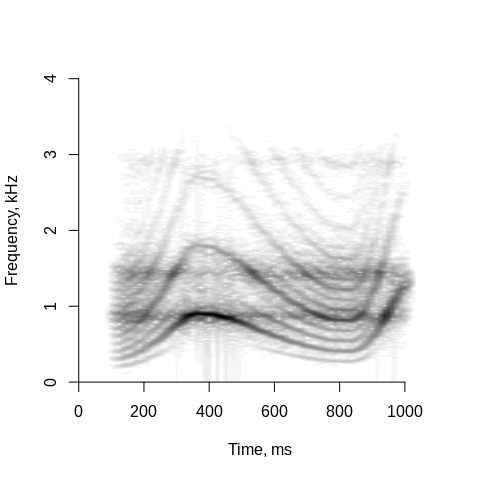``# playme(s1)  # replay as many times as needed w/o re-synthesizing the sound``

The contour of f0 is determined by our pitch anchors, so we can calculate the true median pitch:

``````true_pitch = getSmoothContour(anchors = list(time = c(0, .3, .8, 1),
value = c(300, 900, 400, 1300)),
len = 1000)  # any length will do
median(true_pitch)  # 633 Hz``````
``##  633.2559``

## 2.1 Basic principles

At the heart of acoustic analysis with soundgen is the short-time Fourier transform (STFT): we look at one short segment of sound at a time (one STFT frame), analyze its spectrum using Fast Fourier Transform (FFT), and then move on to the next - perhaps overlapping - frame. As the analysis window slides along the signal, STFT shows which frequencies it contains at different points of time. The nuts and bolts of STFT are beyond the scope of this vignette, but they can be found in just about any textbook on phonetics, acoustics, digital signal processing, etc. For a quick R-friendly introduction, see seewave vignette on acoustic analysis.

Putting the spectra of all frames together, we get a spectrogram. `analyze` calls another function from soundgen package, `spectrogram`, to produce a spectrogram and then plot pitch candidates on top of it. `spectrogram` is a modified version of `seewave::spectro` with added routines for removing background noise, controlling contrast and brightness, adding median smoothing, etc (see the examples in `?spectrogram`). To analyze a sound with default settings and plot its spectrogram, all we need to specify is its sampling rate (the default in soundgen is 16000 Hz):

``a1 = analyze(s1, samplingRate = 16000, plot = TRUE, ylim = c(0, 4))``
``## Scale not specified. Assuming that max amplitude is 1``
``````# summary(a1)  # many acoustic predictors measured for each STFT frame
median(true_pitch)  # true value, as synthesized above``````
``##  633.2559``
``median(a1\$pitch, na.rm = TRUE)  # our estimate``
``##  433.6764``
``````# Pitch postprocessing is stochastic (see below), so the contour may vary.
# Many candidates are off target, mainly b/c of misleading subharmonics.``````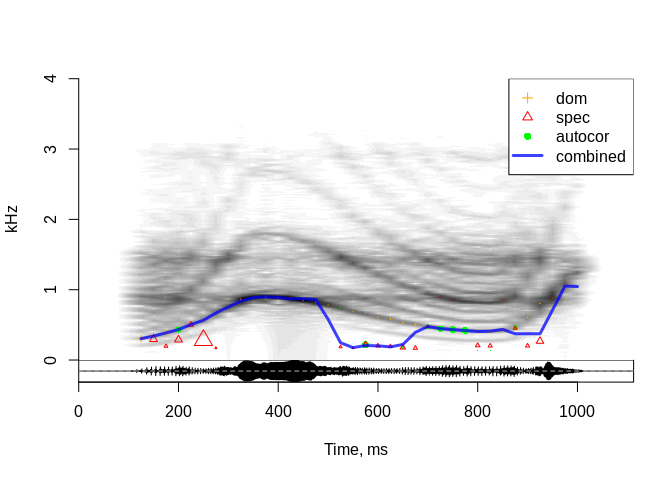There are several key parameters that control the behavior of STFT and affect all extracted acoustic variables. The same parameters serve as arguments to `spectrogram`. As a result, you can immediately see what frame-by-frame input you have fed into the algorithm for acoustic analysis by visually inspecting the produced spectrogram. If you can hear f0, but can’t see individual harmonics in the spectrogram, the pitch tracker probably will not see them, either, and will therefore fail to detect f0 correctly. The first remedy is thus to adjust STFT settings, using the spectrogram for visual feedback:

• `windowLength`: the length of sliding STFT window. Longer windows (e.g., 40 - 50 ms) improve frequency resolution at the expense of time resolution, so they are good for detecting relatively low, slowly changing f0, as in human moans or grunts. Shorter windows (e.g., 5 - 10 ms) improve time resolution at the expense of frequency resolution, so they are good for visualizing formants or tracking high-frequency, rapidly changing f0 as in bird chirps or dolphin whistles.
• `step`: the step of sliding STFT window. For example, if `windowLength = 50` and `step = 25`, each time we move the analysis frame, there is a 50% overlap with the previous frame. This introduces redundancy into the analysis, but it also - to some limited extent - improves time resolution while maintaining relatively high frequency resolution. The main cost of small steps (large overlap) is processing time, but very large overlap is not always desirable, even when processing time is not an issue. If some audio segments are problematic (e.g., very noisy), pitch contour may actually be more accurate with relatively large steps and more smoothing. It is therefore best to check the results with different steps and/or run formal optimization (remember to adjust smoothing and other postprocessing parameters together with STFT settings).
• `wn`: the type of windowing function used to taper the analysis frame during STFT. In practice the windowing function doesn’t seem to have a major effect on the result, as long as you choose something reasonable like gaussian, hanning, or bartlett.
• `zp`: zero-padding. You can use a short STFT window and improve its frequency resolution by padding each frame with zeroes. This is a computational trick that - again, to some limited extent - improves frequency resolution while maintaining relatively high time resolution.
• `silence`: frames with root mean square (RMS) amplitude below silence threshold are not analyzed at all. Quiet frames are harder to analyze, because their signal-to-noise ratio is lower. As a result, we want to strike a good balance. Setting `silence` too low (close to 0) produces a lot of garbage, as the algorithm tries to analyze frames that are essentially just background noise without any signal. Setting `silence` too high (close to 1) excludes too many perfectly good frames, misrepresenting the signal. In soundgen `silence` is dynamically updated: it can never be lower than specified, but it may be raised to the minimum root mean square amplitude of all frames, if this minimum is higher than `silence`. This ensures that empty frames are not analyzed in recordings with unusually high levels of steady background noise (e.g., microphone hiss).

## 2.2 Basic spectral descriptives

Apart from pitch tracking, `analyze` calculates and returns several acoustic characteristics from each non-silent STFT frame:

• `time`: time of the middle of each frame (ms)
• `duration`: total duration (s)
• `duration_noSilence`: duration from the beginning of the first non-silent STFT frame to the end of the last non-silent STFT frame, s (NB: depends strongly on `windowLength` and `silence` settings)
• `ampl`: root mean square of amplitude per frame, calculated as `sqrt(mean(frame ^ 2))`
• `amplVoiced`: the same as `ampl` for voiced frames and `NA` for unvoiced frames
• `dom`: lowest dominant frequency band (Hz) (see “Pitch tracking methods / Dominant frequency”)
• `entropy`: Weiner entropy of the spectrum of the current frame. Close to 0: pure tone or tonal sound with nearly all energy in harmonics; close to 1: white noise
• `f1_freq`, `f1_width`, …: the frequency and bandwidth of the first `nFormants` formants per STFT frame, as calculated by `phonTools::findformants` with default settings
• `harmonics`: the amount of energy in upper harmonics, namely the ratio of total spectral energy above 1.25 x f0 to the total spectral energy below 1.25 x f0 (dB)
• `HNR`: harmonics-to-noise ratio (dB), a measure of harmonicity returned by `soundgen:::getPitchAutocor()` (see “Pitch tracking methods / Autocorrelation”). If HNR = 0 dB, there is as much energy in harmonics as in noise
• `loudness`: subjective loudness in sone, assuming a certain sound pressure level (takes into account the energy in different frequency bands as well as the sensitivity of human ears to different frequencies); see `getLoudness()` and section on Loudness below for details
• `medianFreq`: 50th quantile of the frame’s spectrum
• `peakFreq`: the frequency with maximum spectral energy (Hz)
• `peakFreqCut`: the frequency with maximum spectral energy below `cutFreq` (Hz)
• `quartile25`, `quartile50`, `quartile75`: the 25th, 50th, and 75th quantiles of the spectrum below `cutFreq` (Hz)
• `specCentroid`: the center of gravity of the frame’s spectrum, first spectral moment (Hz)
• `specCentroidCut`: the center of gravity of the frame’s spectrum below cutFreq
• `specSlope`: the slope of linear regression fit to the spectrum below `cutFreq`
• `voiced`: is the current STFT frame voiced? TRUE / FALSE

## 2.3 Custom spectral descriptives

The function `soundgen::analyze` returns a few spectral descriptives that make sense for nonverbal vocalizations, but additional predictors may be useful for other applications (bird songs, non-biological sounds, etc.). One way to obtain extra predictors is to add the necessary code to the internal function `soundgen:::analyzeFrame()` and to `soundgen::analyze()`. If you want deltas, they can be extracted directly from the output of `analyze(..., summary = FALSE)`. But in many cases the easiest solution may be to just extract the spectra and then process them manually, without calling `analyze()`. In fact, many popular spectral descriptors are mathematically trivial to derive - all you need is the spectrum for each STFT frame, or perhaps even the average spectrum of the entire sound. Here is how you can get these spectra.

For the average spectrum of an entire sound, go no further than `seewave::spec` or `seewave::meanspec`:

``````spec = seewave::spec(s1, f = 16000, plot = FALSE)  # FFT of the entire sound
avSpec = seewave::meanspec(s1, f = 16000, plot = FALSE)  # STFT followed by averaging
# either way, you get a dataframe with two columns: frequencies and their strength
``````##            x            y
## [1,] 0.00000 9.717651e-05
## [2,] 0.03125 1.542056e-04
## [3,] 0.06250 2.438498e-04
## [4,] 0.09375 4.312115e-04
## [5,] 0.12500 5.819006e-04
## [6,] 0.15625 6.976463e-04``````

If you are interested in how the spectrum changes over time, extract frame-by-frame spectra - for example, with `spectrogram(..., output = 'original')`:

``````spgm = spectrogram(s1, samplingRate = 16000, output = 'original', plot = FALSE)
# rownames give you frequencies in KHz, colnames are time stamps in ms
str(spgm)``````
``````##  num [1:400, 1:77] 4.61e-04 3.57e-04 1.78e-04 1.09e-04 7.31e-05 ...
##  - attr(*, "dimnames")=List of 2
##   ..\$ : chr [1:400] "0.005" "0.0250250626566416" "0.0450501253132832" "0.0650751879699248" ...
##   ..\$ : chr [1:77] "0" "15" "30" "45" ...``````

Let’s say you are working with frame-by-frame spectra and want to calculate skewness, the 66.6th percentile, and the ratio of energy above/below 500 Hz. Before you go hunting for a piece of software that returns exactly those descriptors, consider this. Once you have normalized the spectrum to add up to 1, it basically becomes a probability density function (pdf), so you can summarize it in the same way as you would any other distribution of a random variable. Look up the formulas you need and just do the raw math:

``````# Transform spectrum to pdf (all columns should sum to 1):
spgm_norm = apply(spgm, 2, function(x) x / sum(x))
# Set up a dataframe to store the output
out = data.frame(skew = rep(NA, ncol(spgm)),
quantile66 = NA,
ratio500 = NA)
# Process each STFT frame
for (i in 1:ncol(spgm_norm)) {
# Absolute spectrum for this frame
df = data.frame(
freq = as.numeric(rownames(spgm_norm)),  # frequency (kHz)
d = spgm_norm[, i]                       # density
)
# plot(df, type = 'l')

# Skewness (see https://en.wikipedia.org/wiki/Central_moment)
m = sum(df\$freq * df\$d)  # spectral centroid, kHz
out\$skew[i] = sum((df\$freq - m)^3 * df\$d)

# 66.6th percentile (2/3 of density below this frequency)
out\$quantile66[i] = df\$freq[min(which(cumsum(df\$d) >= 2/3))]  # in kHz

# Energy above/below 500 Hz
out\$ratio500[i] = sum(df\$d[df\$freq >= .5]) / sum(df\$d[df\$freq < .5])
}``````
``````## Warning in min(which(cumsum(df\$d) >= 2/3)): no non-missing arguments to min;
## returning Inf``````
``summary(out)``
``````##       skew            quantile66         ratio500
##  Min.   : 0.04044   Min.   :0.02503   Min.   :  0.0016
##  1st Qu.: 0.24907   1st Qu.:0.90613   1st Qu.: 13.5314
##  Median : 0.62426   Median :1.12640   Median : 34.0669
##  Mean   : 1.52400   Mean   :1.00309   Mean   : 81.9872
##  3rd Qu.: 1.16263   3rd Qu.:1.24655   3rd Qu.:114.1721
##  Max.   :13.74199   Max.   :1.58698   Max.   :583.3943
##  NA's   :1          NA's   :1         NA's   :1``````

If you need to do this analysis repeatedly, just wrap the code into your own function that takes a wav file as input and returns all these spectral descriptives. You can also save the actual spectra of different sound files and add them up to obtain an average spectrum across multiple sound files, work with cochleograms instead of raw spectra (check out `tuneR::melfcc`), etc. Be your own boss!

## 2.4 Loudness

The digital representation of a sound is a long vector of numbers on some arbitrary scale, say [-1, 1]. Values further from zero correspond to a higher amplitude - in physical terms, to greater pertubations of sound pressure level caused by the propagating sound wave. A smoothed line following peak amplitude values is known as an amplitude envelope. However, there is no simple correspondence between the absolute height of amplitude peaks and the subjectively experienced loudness of the corresponding sound. A commonly reported measure of sound intensity is its root mean square (RMS) amplitude, which takes into account the average value of sound pressure, and not only the height of peaks. More sophisticated estimates of loudness also take into account the relative sensitivity of human hearing to different frequencies, masking of adjacent tones in the time and frequency domains, etc.

To illustrate the differences between these estimates, let’s look at a pure tone sweeping with fixed absolute amplitude from 100 to 4000 Hz over 2 s:

``````dur = 2  # 2 s duration
samplingRate = 16000
f0 = seq(100, 8000, length.out = samplingRate * dur)
sweep = sin(2 * pi * cumsum(f0) / samplingRate)
# playme(sweep)
# spectrogram(sweep, 16000)
# plot(sweep, type = 'l')``````

Smoothed absolute amplitude envelope (flat):

``seewave::env(sweep, f = samplingRate, envt = 'abs', msmooth=c(50, 0))``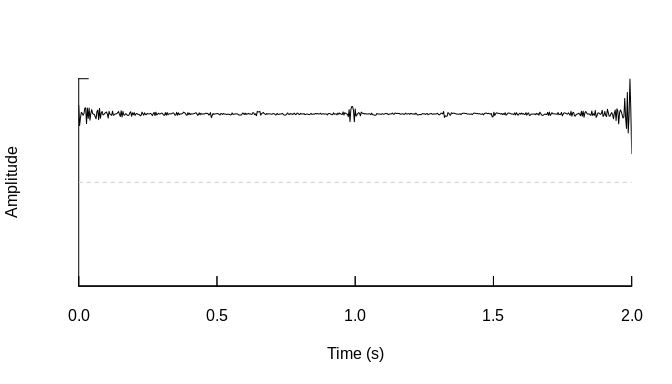RMS amplitude per STFT frame, as returned by `analyze()`, column “ampl”:

``a = analyze(sweep, samplingRate = samplingRate, pitchMethods = NULL, plot = FALSE)``
``## Scale not specified. Assuming that max amplitude is 1``
``plot(seq(0, dur, length.out = length(a\$ampl)), a\$ampl, type = 'b', xlab= 'Time, s')``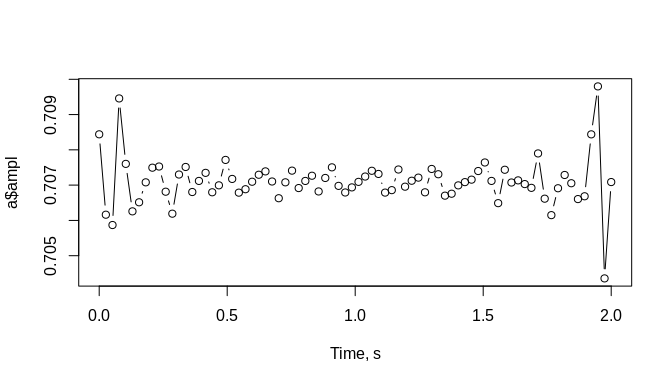An estimate of subjectively experienced loudness in sone, column “loudness”:

``plot(seq(0, dur, length.out = length(a\$loudness)), a\$loudness, type = 'b', xlab= 'Time, s')``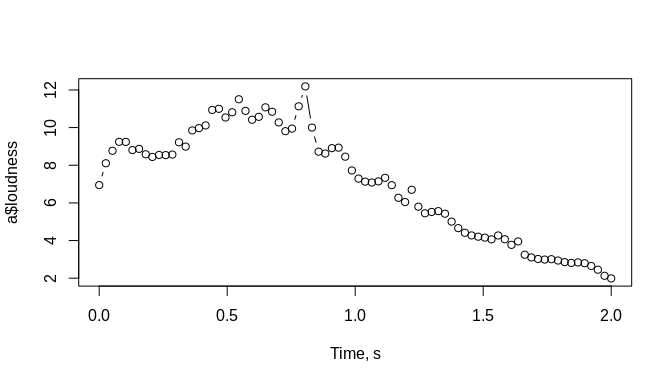Soundgen also has a dedicated function for calculating the loudness and plotting the output, `getLoudness()`. Loudness values are overlaid on the spectrogram - observe how the loudness peaks as f0 reaches about 2-3 kHz and then drops. The absolute values in sone are only an approximation, since they are dictated by the playback device (e.g. your headphones), but the change of loudness within one sound, or across different sounds analyzed with the same settings, is informative.

``l = getLoudness(sweep, samplingRate = samplingRate)``
``````## Warning in getLoudness(sweep, samplingRate = samplingRate): Scale not specified.
## Assuming that max amplitude is 1``````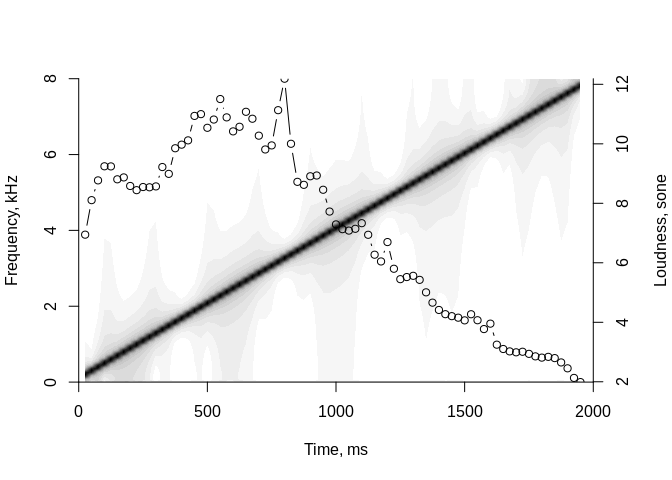## 2.5 Pitch tracking

If you look at the source code of `soundgen::analyze()` and embedded functions, you will see that almost all of this code deals with a single acoustic characteristic: fundamental frequency (f0) or its perceptual equivalent, pitch. That’s because pitch is both highly salient to listeners and notoriously difficult to measure accurately. The approach followed by soundgen’s pitch tracker is to use several different estimates of f0, each of which is better suited to certain types of sounds. You can use any pitch tracker individually, but their output is also automatically integrated and postprocessed so as to generate the best overall estimate of frame-by-frame pitch. There are four currently implemented classes of pitch estimates in soundgen: autocorrelation, lowest dominant frequency, cepstrum, and spectrum (ratios of harmonics). These four methods of pitch estimation are not treated as completely independent in soundgen. Autocorrelation is performed first to provide an initial guess at the likely pitch and harmonics-to-noise ratio (HNR) of an STFT frame, and then this information is used to adjust the expectations of the cepstral and spectral algorithms. In particular, if autocorrelation suggests that the pitch is high, confidence in cepstral estimates is attenuated; and if autocorrelation suggests that HNR is low, thresholds for spectral peak detection are raised, making spectral pitch estimates more conservative.

The plot below shows a spectrogram of the sound with overlaid pitch candidates: yellow circles = autocorrelation pitch estimate (`pitchAutocor`), red triangles = spectral pitch estimate (`pitchSpec`), orange crosses = lowest dominant frequency band (`dom`). The size of each point shows the certainty of estimation: smaller points are calculated with lower certainty and have less weight when all candidates are integrated into the final pitch contour (blue line). To specify which pitch tracking methods should be employed, use `pitchMethods`. For example, we can also include cepstral estimates (violet “envelope” symbols):

``````a = analyze(s1, samplingRate = 16000, plot = TRUE, ylim = c(0, 4),
pitchMethods = c('autocor', 'cep', 'dom', 'spec'))``````
``## Scale not specified. Assuming that max amplitude is 1``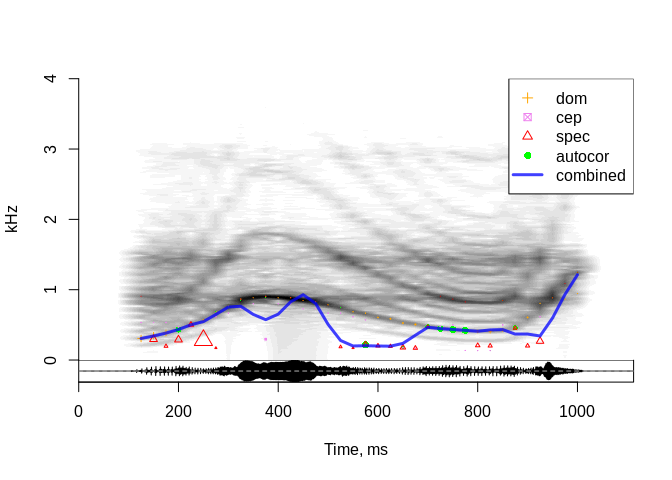### 2.5.1 General settings

`analyze` has a few arguments that affect all methods of pitch tracking:

• `entropyThres`: all non-silent frames are analyzed to produce basic spectral descriptives. However, pitch tracking is both computationally costly and can be misleading if applied to obviously voiceless frames. To define what an “obviously voiceless” frame is, we set some cutoff value of Weiner entropy, above which we don’t want to even try pitch tracking. To disable this feature and track pitch in all non-silent frames, set `entropyThres` to 1.
• `pitchFloor`, `pitchCeiling`: absolute thresholds for pitch candidates. No values outside these bounds will be considered.
• `priorMean` and `priorSD` specify the mean and sd of gamma distribution describing our prior knowledge about the most likely pitch values. The prior works by scaling the certainties associated with particular pitch candidates. If you are working with a single type of sound, such as speech by a male speaker or cricket sounds, specifying a strong prior can greatly improve the quality of the resulting pitch contour. When batch-processing a large number of sounds with `analyzeFolder()`, the recommended approach is to set a vague, but still mildly informative prior. `priorMean` is specified in Hz, but the expected deviation from this typical value is calculated on a musical scale, so `priorSD` is in semitones. For example, if we expect f0 values of about 300 Hz plus-minus half an octave (6 semitones), a prior can be defined as `priorMean = 300, priorSD = 6`. For convenience, the prior can be plotted with `getPrior`:
``````par(mfrow = c(1, 2))
# default prior in soundgen
getPrior(priorMean = 300, priorSD = 6)
# narrow peak at 2 kHz
getPrior(priorMean = 2000, priorSD = 1)
par(mfrow = c(1, 1))``````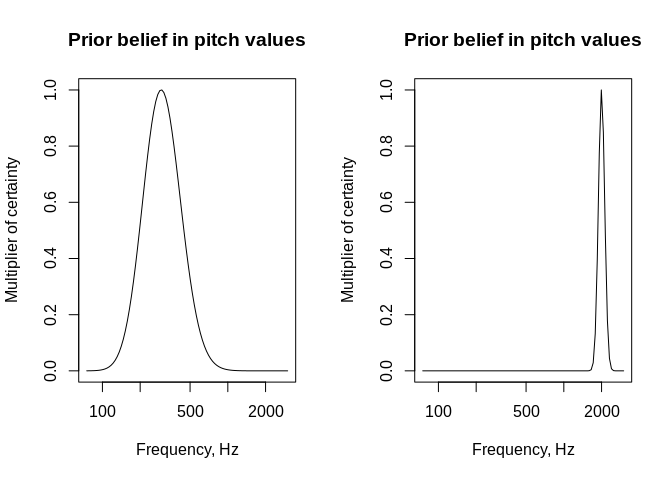TIP The final pitch contour can still pass through low-certainty candidates. You can think of the prior as a soft alternative (or addition) to the inflexible bounds of `pitchFloor` and `pitchCeiling`

• `nCands`: maximum number of pitch candidates to use per method. This only affects `pitchAutocor`, `pitchCep`, and `pitchSpec`. `dom` never returns more than one candidate per frame, since it doesn’t make sense to consider several lowest dominant frequency bands - this simply drags the final pitch contour upwards without improving the accuracy.
• `minVoicedCands`: minimum number of pitch candidates that have to be defined to consider a frame voiced. It defaults to ‘autom’, which means 2 if `dom` is among the candidates and 1 otherwise. The reason is that `dom` is usually defined, even if the frame is clearly voiceless, so we want another pitch candidate in addition to `dom` before we classify the frame as voiced.

### 2.5.2 Pitch tracking methods

Having looked at the general settings, it is time to consider the theoretical principles behind each pitch tracking method, together with arguments to `analyze` that can be used to tweak each one.

#### 2.5.2.1 Autocorrelation

Time domain: pitch by autocorrelation, PRAAT, `pitchAutocor`.

This is an R implementation of the algorithm used in the popular open-source program PRAAT (Boersma, 1993). The basic idea is that a harmonic signal correlates with itself most strongly at a delay equal to the period of its fundamental frequency (f0). Peaks in the autocorrelation function are thus treated as potential pitch candidates. The main trick is to choose an appropriate windowing function and adjust for its own autocorrelation. Compared to other methods implemented in soundgen, pitch estimates based on autocorrelation appear to be particularly accurate for relatively high values of f0. The settings that control `pitchAutocor` are:

• `autocorThres`: voicing threshold, defaults to 0.7. This means that peaks in the autocorrelation function have to be at least 0.7 in height (1 = perfect autocorrelation). A lower threshold produces more false positives (f0 is detected in voiceless, noisy frames), whereas a higher threshold produces more accurate values f0 at the expense of failing to detect f0 in noisier frames.
• `autocorSmooth`: the width of smoothing interval (in bins) for finding peaks in the autocorrelation function. If left NULL, it defaults to 7 for sampling rate 44100 and smaller odd numbers for lower sampling rate.

To use only autocorrelation pitch tracking, but with lower-than-default voicing threshold and more candidates, we can do something like this (prior is disabled so as not to influence the certainties of different pitch candidates):

``````a = analyze(s1, samplingRate = 16000,
plot = TRUE, ylim = c(0, 4), priorMean = NA,
pitchMethods = 'autocor',
autocorThres = .45,
nCands = 3)``````
``## Scale not specified. Assuming that max amplitude is 1``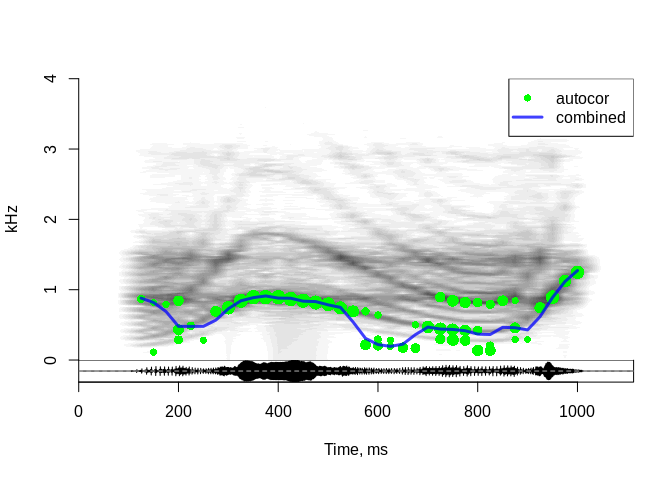#### 2.5.2.2 Dominant frequency

Frequency domain: the lowest dominant frequency band, `dom`.

If the sound is harmonic and relatively noise-free, the spectrum of a frame typically has little energy below f0. It is therefore likely that the first sizable peak in the spectrum is in fact f0, and all we have to do is choose a reasonable threshold. Naturally, there are cases of missing f0 and misleading low-frequency noises. Nevertheless, this simple estimate is often surprisingly accurate, and it may be our best shot when the vocal cords are vibrating in a chaotic fashion (deterministic chaos). For example, sounds such as roars lack clear harmonics but are perceived as voiced, and the lowest dominant frequency band often corresponds to perceived pitch.

The settings that control `dom` are:

• `domThres` (defaults to 0.1, range 0 to 1): to find the lowest dominant frequency band, we look for the lowest frequency with amplitude at least `domThres`. This key setting has to be high enough to exclude accidental low-frequency noises, but low enough not to miss f0. As a result, the optimal level depends a lot on the type of sound analyzed and recording conditions.
• `domSmooth` (defaults to 220 Hz): the width of smoothing interval (Hz) for finding the lowest spectral peak. The idea is that we are less likely to hit upon some accidental spectral noise and find the lowest harmonic (or the lowest spectral band with significant power) if we apply some smoothing to the spectrum of an STFT frame, in this case a moving median.

For the sound we are trying to analyze, we can increase `domSmooth` and/or raise `domThres` to ignore the subharmonics and trace the true pitch contour:

``````a = analyze(s1,
samplingRate = 16000, plot = TRUE, ylim = c(0, 4), priorMean = NA,
pitchMethods = 'dom',
domThres = .1,
domSmooth = 500)``````
``## Scale not specified. Assuming that max amplitude is 1``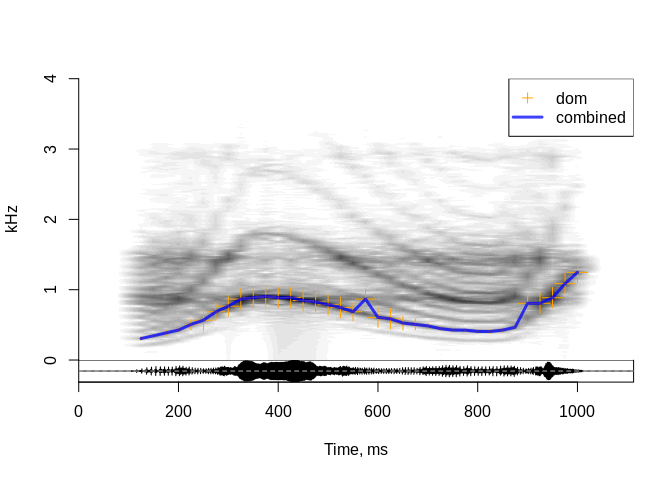#### 2.5.2.3 Cepstrum

Frequency domain: pitch by cepstrum, `pitchCep`.

Cepstrum is the FFT of log-spectrum. It may be a bit challenging to wrap one’s head around, but the main idea is quite simple: just as FFT is a way to find periodicity in a signal, cepstrum is a way to find periodicity in the spectrum. In other words, if the spectrum contains regularly spaced harmonics, its FFT will contain a peak corresponding to this regularity. And since the distance between harmonics equals the fundamental frequency, this cepstral peak gives us f0. Actually, in soundgen the FFT is applied to raw spectrum, not log-spectrum, since it appears to produce better results. Cepstrum is not very useful when f0 is so high that the spectrum contains only a few harmonics, so soundgen automatically discounts the contribution of high-frequency cepstral estimates. Cepstral pitch tracking is disabled by default, since this method is both slower and less robust for human non-linguistic vocalizations. Depending on the type of analyzed audio, however, both the accuracy of different pitch tracking methods and their optimal parameters may change (see the section on optimization below).

The settings that control `pitchCep` are:

• `cepThres`: voicing threshold (defaults to 0.3).
• `cepSmooth`: the width of smoothing interval (in Hz) for finding peaks in the cepstrum. If left NULL, it defaults to 31 for sampling rate 44100 and smaller odd numbers for lower values of sampling rate.
• `cepZp` (defaults to 0): zero-padding of the spectrum used for cepstral pitch detection (points). Zero-padding may improve the precision of cepstral pitch detection, but it also slows down the algorithm.
``````a = analyze(s1,
samplingRate = 16000, plot = TRUE, ylim = c(0, 4), priorMean = NA,
pitchMethods = 'cep',
cepThres = .3,
cepSmooth = 3,
nCands = 2)``````
``## Scale not specified. Assuming that max amplitude is 1``
``````## Warning in analyze(s1, samplingRate = 16000, plot = TRUE, ylim = c(0, 4), :
## cepSmooth should be between 10 and 1000; resetting to 400``````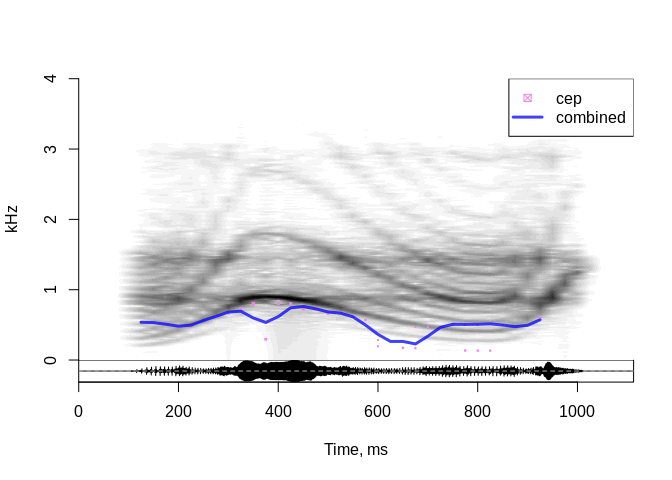#### 2.5.2.4 Spectrum

Frequency domain: ratios of harmonics, BaNa, `pitchSpec`.

All harmonics are multiples of the fundamental frequency. The ratio of two neighboring harmonics is thus predictably related to their rank relative to f0. For example, `(3 * f0) / (2 * f0) = 1.5`, so if we find two harmonics in the spectrum that have a ratio of exactly 1.5, it is likely that these are the first two harmonics, making it possible to calculate f0 (Ba et al., 2012). This is the principle behind the spectral pitch estimate in soundgen, which seems to be particularly useful for noisy, relatively low-pitched sounds.

The settings that control `pitchSpec` are:

• `specThres` (0 to 1, defaults to 0.3): voicing threshold for pitch candidates suggested by the spectral method. The scale is 0 to 1, as usual, but it is the result of a rather arbitrary normalization. The “strength” of spectral pitch candidates is basically calculated as a sigmoid function of the number of harmonic ratios that together converge on the same f0 value. Setting `specThres` too low may produce garbage, while setting it too high makes the spectral method excessively conservative.
• `specPeak` (0 to 1, defaults to 0.35), `specHNRslope` (0 to Inf, defaults to 0.8): when looking for putative harmonics in the spectrum, the threshold for peak detection is calculated as `specPeak * (1 - HNR * specHNRslope)`. For noisy sounds the threshold is high to avoid false sumharmonics, while for tonal sounds it is low to catch weak harmonics. If `HNR` (harmonics-to-noise ratio) is not known, say if we have disabled the autocorrelation pitch tracker or if it returns NA for a frame, then the threshold defaults to simply `specPeak`. This key parameter strongly affects how many pitch candidates the spectral method suggests.
• `specSmooth` (0 to Inf, defaults to 150 Hz): the width of window for detecting peaks in the spectrum, in Hz. You may want to adjust it if you are working with sounds with a specific f0 range, especially if it is unusually high or low compared to human sounds.
• `specMerge` (0 to Inf semitones, defaults to 1): pitch candidates within `specMerge` semitones are merged with boosted certainty. Since the idea behind the spectral pitch tracker is that multiple harmonic ratios should converge on the same f0, we have to decide what counts as “the same” f0.
• `specSinglePeakCert`: (0 to 1, defaults to 0.4) if a`pitchSpec` candidate is calculated based on a single harmonic ratio (as opposed to several ratios converging on the same candidate), its weight (certainty) is taken to be `specSinglePeakCert`. This mainly has implications for how much we trust spectral vs. other pitch estimates.
``````a = analyze(s1,
samplingRate = 16000, plot = TRUE, ylim = c(0, 4), priorMean = NA,
pitchMethods = 'spec',
specPeak = .4,
nCands = 2)``````
``## Scale not specified. Assuming that max amplitude is 1``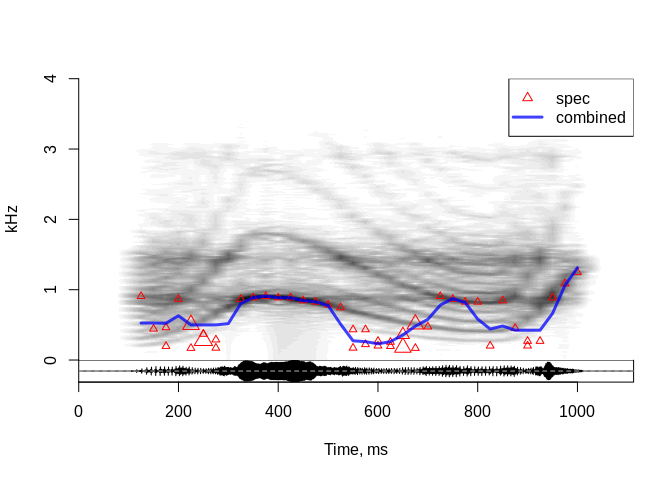TIP As you can guess by now, any pitch tracking method can be tweaked to produce reasonable results for any one particular sound (read: to agree with human intuition). The real trick is to find settings that are accurate on average, across a wide range of sounds and recording conditions. The default settings in `analyze` are the result of optimization against manually verified pitch measurements of a corpus of 260 human non-linguistic vocalizations. For other types of sounds, you will need to perform your own manual tweaking and/or formal optimization.

### 2.5.3 Missing fundamental

The perception of pitch does not depend on the presence of the lowest partial corresponding to the actual fundamental frequency: even if it is removed or masked by low-frequency noise, the pitch remains unchanged. By definition, the “dom” estimate of pitch cannot function when this lowest partial is missing (it works by literally tracking the lowest dominant frequency band). However, the remaining three pitch tracking methods - autocorrelation, cepstrum, and BaNa - have no problem dealing with a missing fundamental frequency because they take the entire spectrum into account, not only the lowest partial.

A sound with four partials at 300 Hz (f0), 600 Hz, 900 Hz, and 1200 Hz:

``````s_withf0 = soundgen(sylLen = 600, pitch = 300,
rolloffExact = c(1, 1, 1, 1), formants = NULL, lipRad = 0)
# playme(s_withf0)
seewave::meanspec(s_withf0, f = 16000, dB = 'max0', flim = c(0, 3))``````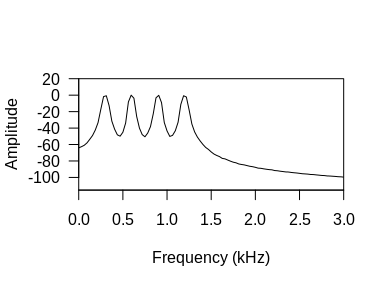The pitch is tracked correctly:

``````a_withf0 = analyze(s_withf0, 16000, pitchMethods = c('autocor', 'cep', 'spec'),
ylim = c(0, 3), dynamicRange = 60)``````
``## Scale not specified. Assuming that max amplitude is 1``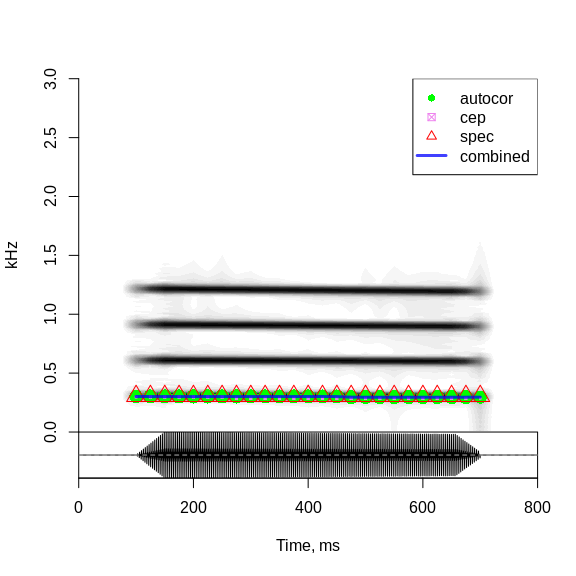The same sound, but without the first partial (f0).

``````s_withoutf0 = soundgen(sylLen = 600, pitch = 300,
rolloffExact = c(0, 1, 1, 1), formants = NULL, lipRad = 0)
# playme(s_withoutf0)  # you can clearly hear the difference
seewave::meanspec(s_withoutf0, f = 16000, dB = 'max0', flim = c(0, 3))``````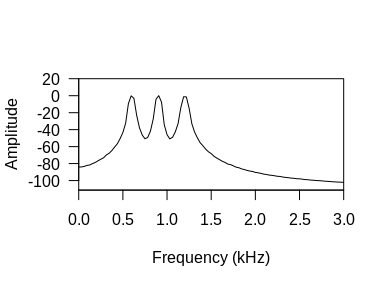Again, no problem with pitch tracking, although now the pitch contour is following a partial that is no longer there:

``````a_withoutf0 = analyze(s_withoutf0, 16000, pitchMethods = c('autocor', 'cep', 'spec'),
ylim = c(0, 3), dynamicRange = 60)``````
``## Scale not specified. Assuming that max amplitude is 1``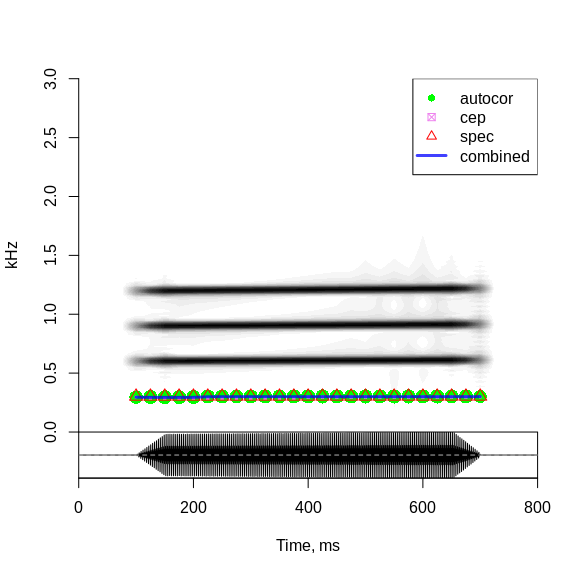The implications are as follows: if the lower part of your signal is degraded (wind noise, an engine running, somebody else talking in the background, etc.), you can apply a high-pass filter to remove low frequencies. Even if you filter out the first partial by doing so, pitch tracking is not going to suffer. BUT: do NOT use the “dom” pitch estimate if the f0 is either filtered out or invisible because of noise!

## 2.6 Postprocessing of pitch contour

Pitch postprocessing in soundgen includes a whole battery of distinct operations through which the pitch candidates generated by one or more tracking methods are integrated into the final pitch contour. We will look at them one by one, in the order in which they are performed in `analyze`. But first of all, here is how to disable them all:

``````a = analyze(
s1,
samplingRate = 16000, plot = TRUE, ylim = c(0, 4), priorMean = NA,
shortestSyl = 0, shortestPause = 0,  # any length of voiced fragments
interpolWin = 0,        # don't interpolate missing f0 values
pathfinding = 'none',   # don't look for optimal path through candidates
snakeStep = 0,          # don't run the snake
smooth = 0              # don't run median smoothing
)       ``````
``## Scale not specified. Assuming that max amplitude is 1``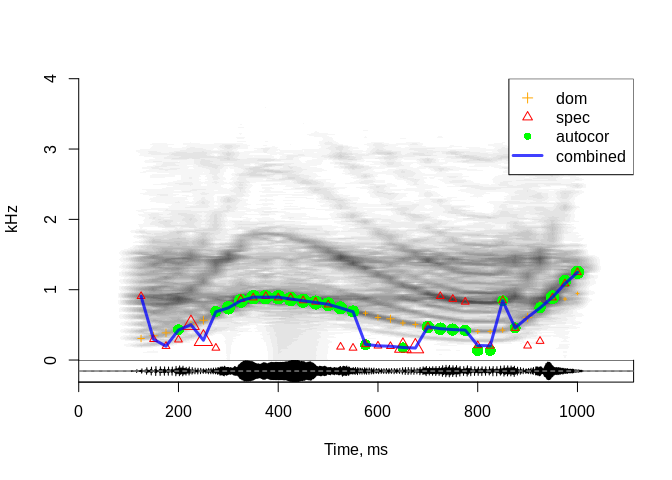When the sound is not too tricky and enough pitch candidates are available, postprocessing actually makes little difference. In terms of the accuracy of median estimate of f0, you are likely to get a good result even with postprocessing is completely disabled. However, if you are interested in the actual intonation contours, not just the global average, postprocessing can help a lot.

### 2.6.1 Continuous voiced fragments

It often makes sense to make assumptions about the possible temporal structure of voiced fragments, such as their minimum expected length (`shortestSyl`) and spacing (`shortestPause`). If these two parameters are positive numbers, the first stage of postprocessing is to divide the sound into continuous voiced fragments that satisfy these assumptions. The default minimum length of a voiced fragment is a single STFT frame. If `shortestSyl` is longer than a single frame, then we need at least two adjacent voiced frames to start a new voiced fragment. A single voiced frame surrounded by unvoiced frames then gets discarded (assumed to be unvoiced). If two voiced fragments are separated by less than `shortestPause`, they are merged. What this means is simply that they are processed as a single syllable by `pathfinder()` (see below). No interpolation takes place at this stage.

The next few blocks of postprocessing are performed by an internal function, `soundgen:::pathfinder()`. Its input is a matrix of pitch candidates for each frame of a single voiced syllable, usually with multiple candidates per frame. Each candidate is also associated with a different certainty. We want to find a good path through these candidates - that is, a pitch contour that both passes close to the strongest candidates and minimizes pitch jumps, producing a relatively smooth contour. The simplest first approximation is to take a mean of all pitch candidates per frame weighted by their certainty - the “center of gravity” of pitch candidates - and for each frame to select the candidate that lies closest to this center of gravity. This initial guess at a reasonable path may or may not be processed further, depending on the settings described below.

### 2.6.2 Interpolation

To make sure we have at least one pitch candidate for every frame in the supposedly continuous voiced fragment, we interpolate to fill in any missing values. The same algorithm also adds new pitch candidates with certainty `interpolCert` if a frame has no pitch candidates within `interpolTol` of the median of the “center of gravity” estimate over plus-minus `interpolWin` frames. The frequency of new candidates is equal to this median. For example, if `interpolTol = 0.05`, new candidates are calculated if there are none within 0.95 to 1.05 times the median over the interpolation window. You can also enable interpolation to fill in unvoiced frames, but without adding new pitch candidates in voiced frames. To do so, set `interpolTol = Inf`.

Here is an example (interpolated segments are shown with a dotted line)

``````a1 = analyze(s1, samplingRate = 16000, priorMean = NA,
pitchMethods = 'cep', cepThres = .4, step = 25,
snakeStep = 0, smooth = 0,
interpolWin = 0,   # disable interpolation
pathfinding = 'none',
summary = FALSE,
plot = FALSE)``````
``## Scale not specified. Assuming that max amplitude is 1``
``````a2 = analyze(s1, samplingRate = 16000, priorMean = NA,
pitchMethods = 'cep', cepThres = .4, step = 25,
pathfinding = 'none',
snakeStep = 0, smooth = 0,
summary = FALSE,
plot = FALSE)  ``````
``## Scale not specified. Assuming that max amplitude is 1``
``````plot(a1\$time, a1\$pitch, type = 'l', xlab = 'Time, ms', ylab = 'Pitch, Hz')
points(a2\$time, a2\$pitch, type = 'l', col = 'red', lty = 3)``````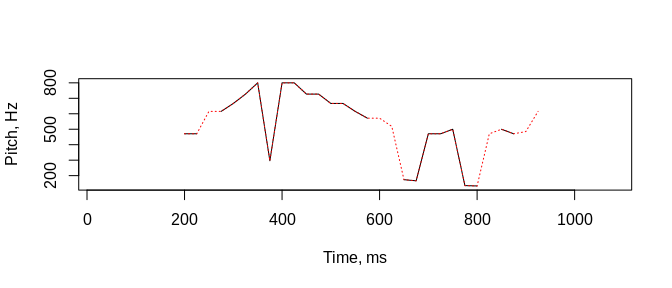### 2.6.3 Pathfinding

The next step after interpolation is pathfinding proper - searching for the optimal path through pitch candidates. If `pathfinding = "none"`, this step is skipped, so we just continue working with the path that lies as close as possible to the (possibly interpolated) center of gravity of pitch candidates. If `pathfinding = "fast"` (the default option), a simple heuristic is employed, in which we walk down the path twice, first left to right and then right to left, trying to minimize the cost measured as a weighted mean of the distance from the center of gravity and the deviation from a smooth contour. The key setting is `certWeight`, which specifies how much we prioritize the certainty of pitch candidates vs. pitch jumps / the internal tension of the resulting pitch curve. Low `certWeight` (close to 0): we are mostly concerned with avoiding rapid pitch fluctuations in our contour. High `certWeight` (close to 1): we mostly pay attention to our certainty in particular pitch candidates. The example below is intended as an illustration of how pathfinding works, so all other types of smoothing are disabled, forcing the final pitch contour to pass strictly through existing candidates.

``````a1 = analyze(s1, samplingRate = 16000, priorMean = NA,
pitchMethods = 'cep', cepThres = .15, nCands = 3,
snakeStep = 0, smooth = 0, interpolTol = Inf,
certWeight = 0,  # minimize pitch jumps
main = 'Minimize jumps',
showLegend = FALSE, osc = FALSE, ylim = c(0, 3))  ``````
``## Scale not specified. Assuming that max amplitude is 1``
``````a2 = analyze(s1, samplingRate = 16000, priorMean = NA,
pitchMethods = 'cep', cepThres = .15, nCands = 3,
snakeStep = 0, smooth = 0, interpolTol = Inf,
certWeight = 1,  # minimize deviation from high-certainty candidates
main = 'Pass through top cand-s',
showLegend = FALSE, osc = FALSE, ylim = c(0, 3))``````
``## Scale not specified. Assuming that max amplitude is 1``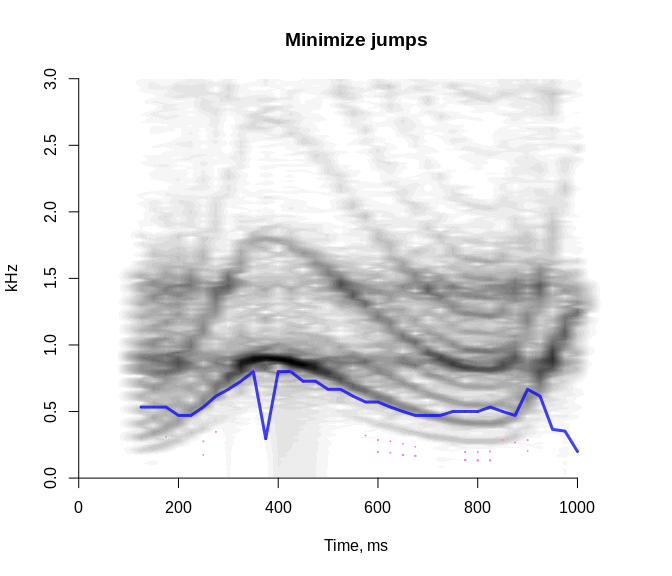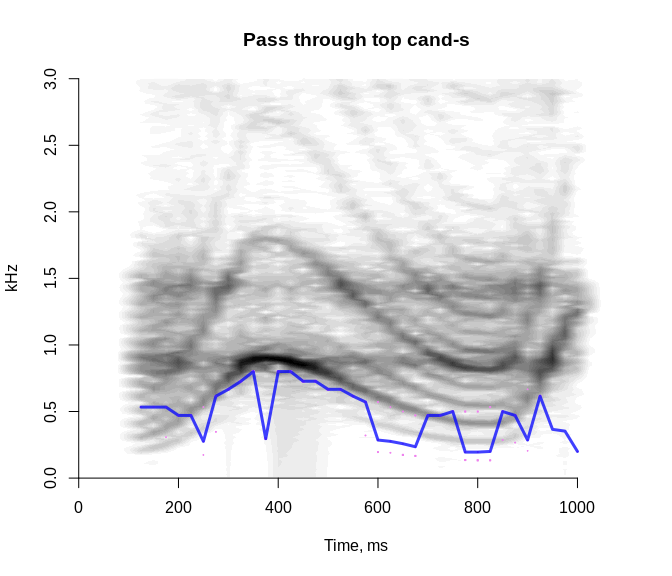The final option is `pathfinding = 'slow'`, which calls `stats::optim(method = 'SANN')` to perform simulated annealing. This is a more powerful algorithm than the simple heuristic in `pathfinding = 'fast'`, but it is called “slow” for a good reason. In case you have plenty of time, it does improve the results, but note that this algorithm is stochastic, so each run may produce different results. Use an additional argument, `annealPars`, to control the algorithm. See `?stats::optim` for more details.

### 2.6.4 Snake

What is here esoterically referred to as the “snake” can be seen as an alternative to the pathfinding algorithms above, although both can also be performed sequentially. Whereas pathfinding attempts to find the best path through existing pitch candidates, the snake wiggles the contour under a weighted combination of (a) elastic forces trying to snap the pitch contour to a straight line and (b) the pull of high-certainty pitch candidates. In a sense the snake is thus a combination of interpolation and pathfinding: like interpolation, it can add new values different from existing candidates, and like pathfinding, it balances the certainty in candidates against the smoothness of the resulting contour.

The only new control parameter in the snake module (apart from `certWeight`) is `snakeStep`, which controls the speed of adaptation (the default is 0.05). The higher it is, the faster the snake “wiggles”. This reduces processing time, but introduces a risk of “overshooting”. If `snakeStep` is too low (close to 0), the snake moves too slowly and may fail to reach its optimal configuration. To disable the snake module, set `snakeStep = NULL`. You can also produce a separate plot of the snake by setting `snakePlot = TRUE`, as in the example below (again, all other postprocessing is disabled to show what the snake alone will do). The zigzagging line is the initial contour (the path through pitch candidates that lie as close as possible to the center of gravity of each frame), the smooth blue line is the pitch contour after running the snake, and the green lines trace the progress of iterative snake adaptation. Note that at `certWeight = 0.1` the snake is heavily biased towards producing a smooth contour, regardless of its distance from high-certainty pitch candidates.

``````a1 = analyze(s1, samplingRate = 16000, plot = FALSE, priorMean = NA,
pitchMethods = 'cep', cepThres = .2, nCands = 2,
pathfinding = 'none', smooth = 0, interpolTol = Inf,
certWeight = 0.1,  # like pathfinding, the snake is affected by certWeight
snakeStep = 0.05, snakePlot = TRUE)``````
``## Scale not specified. Assuming that max amplitude is 1``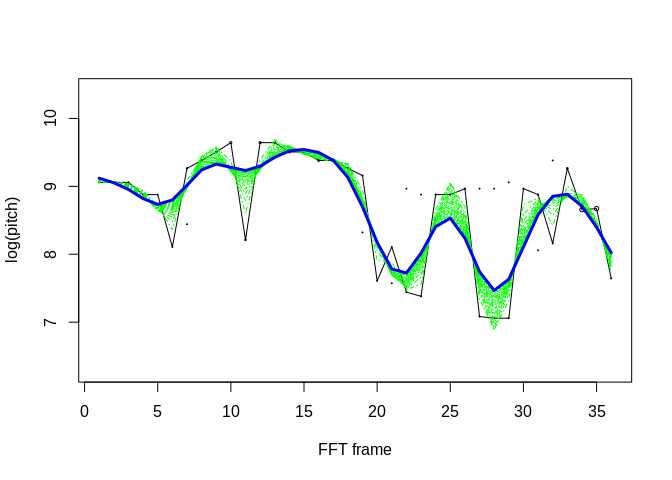TIP Should you use pathfinding, the snake, or both? Pathfinding makes more sense if you want the final contour to pass strictly through existing candidates, say if there are relatively few candidates, most of which are right on target and some completely off. In these conditions the snake will not do much (but not much harm, either). The snake becomes attractive if you have a lot of candidates from different pitch tracking methods, many of which are slightly off and should be averaged. In addition, the more garbage you expect among your pitch candidates, the more you might want to interpolate and apply median smoothing

### 2.6.5 Median smoothing

The final postprocessing stage is median smoothing. It is conceptually similar to interpolation, except that by now there is only a single f0 value left per frame, so we can forget about the multiple candidates and their certainties. It wouldn’t make much sense to apply kernel smoothing to this curve: the snake can usually do this in a smarter way, since it does know about the multiple candidates and their certainties. What we want from the smoothing algorithm is to detect and correct only outliers: the values that stick out from the surrounding frames. The parameters that control this module are `smooth` and `smoothVars`.

If `smooth` is a positive number, contours of the variables in `smoothVars` are smoothed using a customized version of median smoothing. This modifies only the values that deviate considerably from the moving median and preserves all other values (so this is a bit different from applying a moving median or kernel smoothing). `smooth` controls both the tolerated deviance and the size of the window for calculating a moving median. `smooth = 1` (the default) corresponds to a window of ~100 ms and tolerated deviation of ~4 semitones. This smoothing can be applied to any measured value, not only the final pitch contour. The default is `smoothVars = c('pitch', 'dom')`. To turn off the median smoothing, set `smooth = 0` or `smoothVars = NULL`.

``````a1 = analyze(s1, samplingRate = 16000, priorMean = NA,
pitchMethods = 'cep', cepThres = .2, nCands = 2,
pathfinding = 'none', snakeStep = 0, interpolTol = Inf,
smooth = 0,  # no smoothing
summary = FALSE, plot = FALSE)``````
``## Scale not specified. Assuming that max amplitude is 1``
``````a2 = analyze(s1, samplingRate = 16000, priorMean = NA,
pitchMethods = 'cep', cepThres = .2, nCands = 2,
pathfinding = 'none', snakeStep = 0, interpolTol = Inf,
smooth = 1,  # default smoothing
summary = FALSE, plot = FALSE)``````
``## Scale not specified. Assuming that max amplitude is 1``
``````plot(a1\$time, a1\$pitch, type = 'l', xlab = 'Time, ms', ylab = 'Pitch, Hz')
points(a2\$time, a2\$pitch, type = 'l', col = 'red', lty = 3, lwd = 2)``````